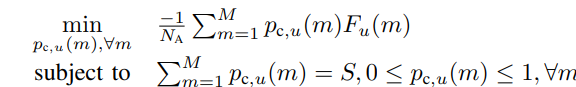# I am not sure why the solution of CVX is transpose

It’s my first time using CVX.
Although I read the documentation, I still confuse why the solution of pc_u looks so weird especially the
matrix size of pc_u

There is my code
for u=1:N
cvx_begin
size(pc_user)
variable pc_u(u,m)
minimize( -(sum(pc_u(u,m).*F_u(u,:)))/numactive)
subject to
sum(pc_u(u,:)) == S
0<=pc_u(:,m)<=1

``````cvx_end
pc_u
``````

endthe value of parameter respectively
N=3 M=10
m had been equal to 10

This might be `variable pc_u(1,M)`. In your above code, you define a new u-by-m variable array by `variable pc_u(u,m)` for every iteration (also u is changing), which might not be what you wanted. If you need to save your solution of every iteration to an array, you can define another M-by-N array outside the loop rather than to define it CVX variable.

1 Like